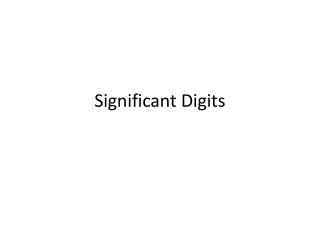DownloadDownload PresentationSignificant Digits

# Significant Digits

Download Presentation## Significant Digits

- - - - - - - - - - - - - - - - - - - - - - - - - - - E N D - - - - - - - - - - - - - - - - - - - - - - - - - - -
##### Presentation Transcript

1. Significant Digits

2. Other ways of saying it… • Significant Digits • Significant Figures • Sigfigs • Sigdigs

3. The problem… • Numbers are perfect and exact • Measurements are neither perfect nor exact • Accuracy = closeness to correct value • Accuracy itself is not a number, but a description • Precision = how close multiple measurements came to one another • Precision can also describe the number of sigfigs • The precision of an answer is not the same as the accuracy of an answer.

4. Precision vs. Accuracy Accurate, but not precise Precise, but not accurate

5. Precision vs. Accuracy Accurate and precise Neither accurate nor precise

6. Numerical example: • Let’s say a table has a correct height of 0.68215 m • 4 groups measure the table several times… • 0.7 m, 0.6 m, 0.7 m • 0.91521 m, 0.91525 m, 0.91528 m • 0.6822 m, 0.6821 m, 0.6822 m • 2 m, 3 m, 1 m

7. How ALL measurements are to be made • Read your measurements to all known values plus one estimated value • Check your understanding

8. A P resent bsent

9. Counting Significant Digits in somebody else’s measurement • If decimal point is Present • Count all digits from left to right starting with first nonzero number • If decimal point is Absent • Count all digits from right to left starting with first nonzero number

10. A P resent bsent

11. Count the significant digits • 0.000275 • 3 digits • 1.0052 • 5 digits • 270 • 2 digit • 25300 • 3 digits A P

12. Typical Example of using and following significant digits through a calculation • Read the measurement from the BOTTOM of the meniscus • lazy answer: 21 mL • better answer: 20.6 mL • best answer: 20.65 mL

13. Density is mass divided by volume. The mass of 20.65 mL of acetone is measured to be 16.3 g. Calculate the density of acetone. • But our calculation cannot be more precise than our measurements. (Round to ___ digits) • D = 0.789 g/mL

14. Adding with significant digits • Add these masses: 0.265 g, 0.1820 g, 12.05 g • 00.265 g • 00.1820 g • 12.05 g • ============ • 12.497 g • (but the least precise measurement forces us to round to the hundredths place) • 12.50 g

15. Calculating error and percent error • Density of lead is measured to be 10.9 g/cm3. • Actual, accept, or correct value of the density of lead is 11.34 g/cm3. • Error = experimental value – accepted value

16. Summary • When making a measurement, report all known numbers, plus one estimated digit. • Counting significant digits with a decimal point left to right • Counting significant digits without a decimal point right to left • Multiplying or dividing with sigfigs, your result has no more sigfigs than the number with the least sigfigs in the problem. • Adding or subtracting with sigfigs, your answer has no more decimal places than the number with the fewest decimal places in the problem. • Error = exp. value – measured value • % error = absolute value of error divided by accepted value * 100.